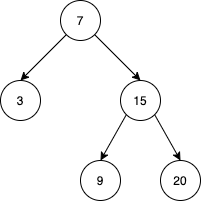Formatted question description: https://leetcode.ca/all/1586.html

# 1586. Binary Search Tree Iterator II

Medium

## Description

Implement the BSTIterator class that represents an iterator over the in-order traversal of a binary search tree (BST):

• BSTIterator(TreeNode root) Initializes an object of the BSTIterator class. The root of the BST is given as part of the constructor. The pointer should be initialized to a non-existent number smaller than any element in the BST.
• boolean hasNext() Returns true if there exists a number in the traversal to the right of the pointer, otherwise returns false.
• int next() Moves the pointer to the right, then returns the number at the pointer.
• boolean hasPrev() Returns true if there exists a number in the traversal to the left of the pointer, otherwise returns false.
• int prev() Moves the pointer to the left, then returns the number at the pointer.

Notice that by initializing the pointer to a non-existent smallest number, the first call to next() will return the smallest element in the BST.

You may assume that next() and prev() calls will always be valid. That is, there will be at least a next/previous number in the in-order traversal when next()/prev() is called.

Follow up: Could you solve the problem without precalculating the values of the tree?

Example 1:Input
["BSTIterator", "next", "next", "prev", "next", "hasNext", "next", "next", "next", "hasNext", "hasPrev", "prev", "prev"]
[[[7, 3, 15, null, null, 9, 20]], [null], [null], [null], [null], [null], [null], [null], [null], [null], [null], [null], [null]]
Output
[null, 3, 7, 3, 7, true, 9, 15, 20, false, true, 15, 9]

Explanation
// The underlined element is where the pointer currently is.
BSTIterator bSTIterator = new BSTIterator([7, 3, 15, null, null, 9, 20]); // state is   [3, 7, 9, 15, 20]
bSTIterator.next(); // state becomes [3, 7, 9, 15, 20], return 3
bSTIterator.next(); // state becomes [3, 7, 9, 15, 20], return 7
bSTIterator.prev(); // state becomes [3, 7, 9, 15, 20], return 3
bSTIterator.next(); // state becomes [3, 7, 9, 15, 20], return 7
bSTIterator.hasNext(); // return true
bSTIterator.next(); // state becomes [3, 7, 9, 15, 20], return 9
bSTIterator.next(); // state becomes [3, 7, 9, 15, 20], return 15
bSTIterator.next(); // state becomes [3, 7, 9, 15, 20], return 20
bSTIterator.hasNext(); // return false
bSTIterator.hasPrev(); // return true
bSTIterator.prev(); // state becomes [3, 7, 9, 15, 20], return 15
bSTIterator.prev(); // state becomes [3, 7, 9, 15, 20], return 9


Constraints:

• The number of nodes in the tree is in the range [1, 10^5].
• 0 <= Node.val <= 10^6
• At most 10^5 calls will be made to hasNext, next, hasPrev, and prev.

## Solution

Do in-order traversal and create a doubly-linked list based on the nodes. Each node’s left points to its predecessor and each node’s right points to its successor. Add a dummyHead before the first node in the in-order traversal.

For hasNext() and hasPrev() operations, check whether the current node’s successor is null or the current node’s predecessor is dummyHead, respectively. If this is the case, return false. Otherwise, return true.

For next() and prev() operations, move to the successor or the predecessor, and return the node’s value after moving.

/**
* Definition for a binary tree node.
* public class TreeNode {
*     int val;
*     TreeNode left;
*     TreeNode right;
*     TreeNode() {}
*     TreeNode(int val) { this.val = val; }
*     TreeNode(int val, TreeNode left, TreeNode right) {
*         this.val = val;
*         this.left = left;
*         this.right = right;
*     }
* }
*/
class BSTIterator {
TreeNode dummyHead = new TreeNode(0);
TreeNode curr;

public BSTIterator(TreeNode root) {
Deque<TreeNode> stack = new LinkedList<TreeNode>();
TreeNode prev = dummyHead, node = root;
while (node != null || !stack.isEmpty()) {
if (node != null) {
stack.push(node);
node = node.left;
} else {
node = stack.pop();
TreeNode visitNode = new TreeNode(node.val);
prev.right = visitNode;
visitNode.left = prev;
prev = visitNode;
node = node.right;
}
}
}

public boolean hasNext() {
return curr.right != null;
}

public int next() {
curr = curr.right;
return curr.val;
}

public boolean hasPrev() {
return curr.left != dummyHead && curr != dummyHead;
}

public int prev() {
curr = curr.left;
return curr.val;
}
}

/**
* Your BSTIterator object will be instantiated and called as such:
* BSTIterator obj = new BSTIterator(root);
* boolean param_1 = obj.hasNext();
* int param_2 = obj.next();
* boolean param_3 = obj.hasPrev();
* int param_4 = obj.prev();
*/


/**
* Definition for a binary tree node.
* public class TreeNode {
*     int val;
*     TreeNode left;
*     TreeNode right;
*     TreeNode() {}
*     TreeNode(int val) { this.val = val; }
*     TreeNode(int val, TreeNode left, TreeNode right) {
*         this.val = val;
*         this.left = left;
*         this.right = right;
*     }
* }
*/
class BSTIterator {
Stack<TreeNode> stack = new Stack<>();
List<TreeNode> list = new ArrayList<>();
int index = -1;

private void pushLeft(TreeNode root) {
while (root != null) {
stack.push(root);
root = root.left;
}
}

private boolean inRange(int i) {
return i >= 0 && i < list.size();
}

public BSTIterator(TreeNode root) {
pushLeft(root);
}

public boolean hasNext() {
if (inRange(index + 1)) {
return true;
}
return !stack.isEmpty();
}

public int next() {
int nextVal = 0;
if (inRange(index + 1)) {
nextVal = list.get(index + 1).val;
} else {
TreeNode next = stack.pop();
pushLeft(next.right);
nextVal = next.val;
}
index++;
return nextVal;
}

public boolean hasPrev() {
return inRange(index - 1);
}

public int prev() {
return list.get(--index).val;
}
}

/**
* Your BSTIterator object will be instantiated and called as such:
* BSTIterator obj = new BSTIterator(root);
* boolean param_1 = obj.hasNext();
* int param_2 = obj.next();
* boolean param_3 = obj.hasPrev();
* int param_4 = obj.prev();
*/